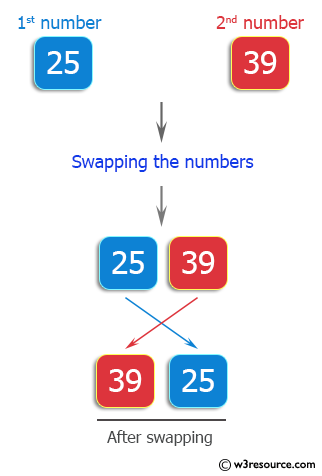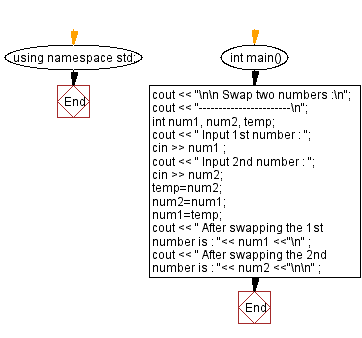﻿ C++ Exercises: Swap two numbers - w3resource

# C++ Exercises: Swap two numbers

## C++ Basic: Exercise-13 with Solution

Write a C++ program that swaps two numbers.

Visual Presentation:Sample Solution :-

C++ Code :

``````#include <iostream> // Including the input-output stream header file

using namespace std; // Using the standard namespace

int main() // Start of the main function
{
cout << "\n\n Swap two numbers :\n"; // Outputting a message indicating swapping of two numbers
cout << "-----------------------\n"; // Outputting a separator line

int num1, num2, temp; // Declaring integer variables num1, num2, and temp for swapping
cout << " Input 1st number : "; // Prompting the user to input the first number
cin >> num1 ; // Taking input for the first number from the user

cout << " Input 2nd number : "; // Prompting the user to input the second number
cin >> num2; // Taking input for the second number from the user

temp = num2; // Storing the value of num2 in a temporary variable temp
num2 = num1; // Assigning the value of num1 to num2
num1 = temp; // Assigning the value of temp (initially num2) to num1 to complete the swap

cout << " After swapping the 1st number is : "<< num1 <<"\n" ; // Displaying the value of num1 after swapping
cout << " After swapping the 2nd number is : "<< num2 <<"\n\n" ; // Displaying the value of num2 after swapping

return 0; // Returning 0 to indicate successful program execution
} // End of the main function
``````

Sample Output:

``` Swap two numbers :
-----------------------
Input 1st number : 25
Input 2nd number : 39
After swapping the 1st number is : 39
After swapping the 2nd number is : 25
```

Flowchart:C++ Code Editor:

What is the difficulty level of this exercise?

﻿